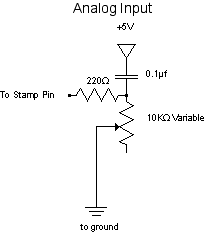Sometimes we need to measure quantities in the real world, make variable changes in a program: for example, how much light there is, how loud is a sound, and so forth. In these cases, we can't use a digital input, but we need to convert the variable condition into the real world to something variable that we can measure. Most commonly, we create a variable voltage. To do this, we make an analog input circuit.Since the Basic Stamp can't actually read varying voltages, we use a resistor-capacitor circuit. In this circuit, we charge the capacitor from one of the pins of the stamp, them measure how long it takes to discharge. The result for us is that the stamp gets a varying number between 0 and 65535 depending on where the maximum resistance of the variable resistor, and where it is set.

The command on the stamp is the RCTIME command, and it looks like this:

`RCTIME pin, STATE, result`

PIN is the stamp pin you are using;
STATE is a pin state (high or low, 1 or 0) that will end the RCTIME command (I always use 1)
RESULT is a word variable that tells the time the capacitor took to discharge

To program the stamp to read this, use this code (assuming you're using stamp pin 1):

```checkPot:

HIGH 1 				'put current out to the capacitor
PAUSE 1 			'hold a millisecond
RCTIME 1,1, potVar		'measure the cap's discharge time
DEBUG ?potVar			'see the value of potVar on the PC screen
GOTO checkPot
```

The number produced in potVar is usually a word-sized variable (0-65535). However, you usually want a byte-sized range of numbers. To convert it, first find out what the variable resistor's maximum value is. Then produce a byte variable from it like this:

byteVar = (potVar / maximum) * 255

This formula is a good rule of thumb, but not an absolute rule. Use whatever conversion gives you the range of numbers you want. For example, if your variable resistor produces a range between 0 and 600, and you want a value between 0 and 10, potVar /60 would work just fine.

Note that because of timing differences, the numbers for the same resistor will be very different on a Basic stamp 2 than on a Basic stamp 2SX!

## Analog Output

The Basic Stamp is not very good at analog output. However, there are a few ways to "fake" it. One way is to set up a digital output circuit and use the PWM command. This uses a technique known as Pulse-width modulation to make the stamp pulse high at varying rates. As the pulse drops off, the voltage goes to zero. The voltage produced is an average of the high pulses and the low (zero). The more you pulse, the more the stamp pin is high,and therefore the closer it is to 5V. The less you pulse, the closer it is to zero.

The PWM command looks like this:

`PWM pin, duty, cycles`

PIN is the stamp pin you're using;
DUTY is a byte-sized variable (0-255) specifying the voltage (0-5V).
CYCLES is a word-sized variable (0-65535) specifying the number of milliseconds to output for. For example, let's say you've got an LED on pin 9, and you want to produce a varying voltage based on the varying number you got from the RCTIME circuit above. You'd need to make the word variable potVar into a byte. Let's say potVar varied between 0 and 10,000. You could do this:

```checkPot:

HIGH 1				'put current out to the capacitor
PAUSE 1 			'hold a millisecond
RCTIME 1,1, potVar		'RCTIME to get a value for potVar
byteVar = (potVar/10000) * 255 	'create byteVar, 0-255, that varies with potVar
PWM 9, byteVar, 10		'pulse-width modulate pin 9, voltage = byteVar, for 10 milliseconds
GOTO checkPot
```

The Stamp is good at producing a variable frequency output, however. This is done using the FREQOUT command. Let's say we want to produce a tone based on the position of a variable resistor. If we're using the program above, we can use the potVar variable to give us a varying frequency:

FREQOUT pin, time, frequency

So for the program above, put a speaker on pin 2, and put this line right after the DEBUG command:

FREQOUT 2, 10, potVar

This will generate a tone for 10 milliseconds on the speaker every time you run it.

The BASIC stamp manual has schematic diagrams for wiring a speaker or amplifier to your stamp. Here's one for an 8-ohm speaker: